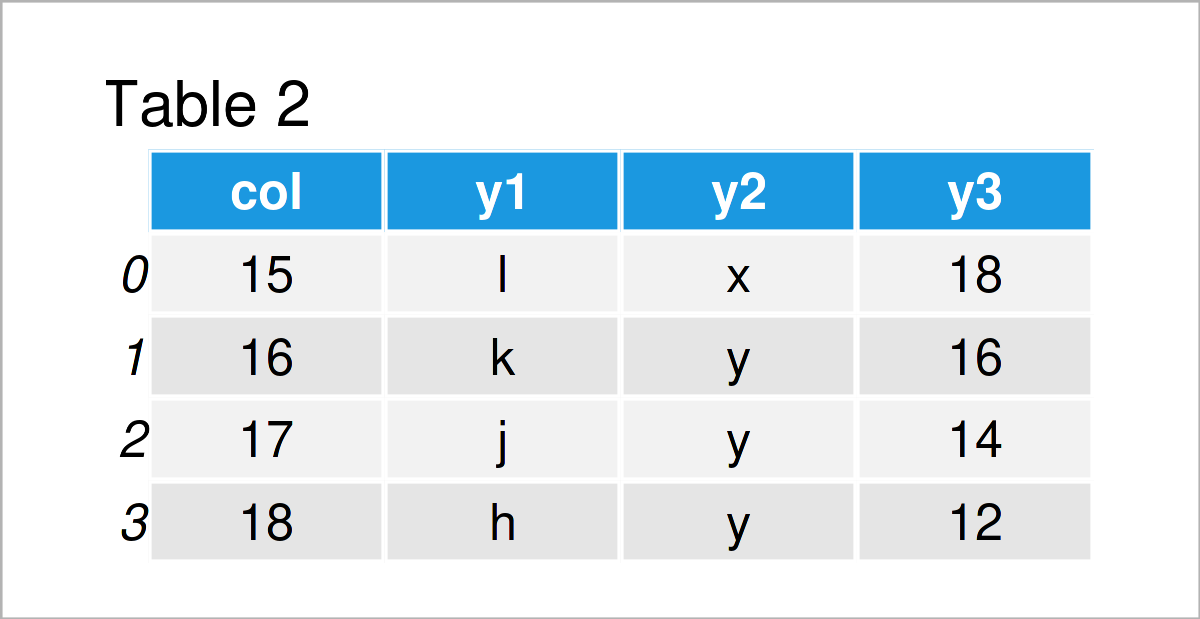# Merge pandas DataFrames based on Particular Column in Python (Example)

In this article, I’ll show how to join two DataFrames based on a shared column in the Python programming language.

The tutorial contains the following information:

Sound good? Let’s dig in:

## Example Data & Libraries

In order to use the functions of the pandas library, we first need to load pandas:

`import pandas as pd                           # Import pandas`

We’ll use the following DataFrames as basement for this Python programming tutorial:

```data1 = pd.DataFrame({"col":range(11, 17),    # Create first pandas DataFrame
"x1":range(12, 1, - 2),
"x2":["a", "b", "c", "d", "e", "f"],
"x3":range(17, 11, - 1)})
print(data1)                                  # Print first pandas DataFrame``````data2 = pd.DataFrame({"col":range(15, 19),    # Create second pandas DataFrame
"y1":["l", "k", "j", "h"],
"y2":["x", "y", "y", "y"],
"y3":range(18, 10, - 2)})
print(data2)                                  # Print second pandas DataFrame```In Tables 1 and 2 it is shown that we have created two different pandas DataFrames containing different values. Both of these DataFrames have the variable “col” in common.

Let’s use this variable to stack and append our DataFrames horizontally.

## Example: Merge Two pandas DataFrames based on Specific Column Using merge() Function

The following code shows how to combine two pandas DataFrames using a particular column as basis.

To achieve this, we have to apply the merge function. Within this function, we have to specify the name of the column we want to use to combine our DataFrames.

Consider the following Python syntax

```data_join = pd.merge(data1,                   # Inner join
data2,
on = "col")
print(data_join)                              # Print merged DataFrame```In Table 3 it is shown that we have created a DataFrame union of our two input data sets.

Note that we have used an inner join to merge our data. However, you may also use different types of joins such as outer joins, left joins, or right joins.

Furthermore, please note that it would also be possible to merge multiple DataFrames based on a single column. Have a look here for more details.

## Video, Further Resources & Summary

Do you need more information on the Python codes of this article? Then you could have a look at the following video on the Statistics Globe YouTube channel. In the video, I’m demonstrating the Python code of this article.

Please accept YouTube cookies to play this video. By accepting you will be accessing content from YouTube, a service provided by an external third party.If you accept this notice, your choice will be saved and the page will refresh.

Furthermore, you may want to read some of the related articles on my homepage. You can find a selection of related posts below.

Summary: You have learned in this tutorial how to combine two DataFrames based on a certain variable in Python. In case you have further questions, don’t hesitate to tell me about it in the comments.

Subscribe to the Statistics Globe Newsletter